# 四、使用直方图计算像素

• 计算图像直方图
• 应用查询表修改图像外观
• 均衡图像直方图
• 反投影直方图来检测特定图像内容
• 使用均值平移算法查找对象
• 使用直方图比较检索相似图像

# 计算图像直方图

## 入门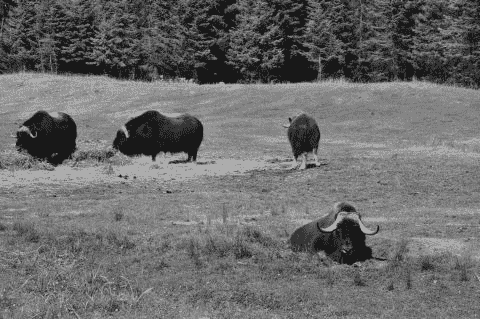## 操作步骤

class Histogram1D {

private:

int histSize;  // number of bins
float hranges; // min and max pixel value
const float* ranges;
int channels;  // only 1 channel used here

public:

Histogram1D() {

// Prepare arguments for 1D histogram
histSize= 256;
hranges= 0.0;
hranges= 255.0;
ranges= hranges;
channels= 0; // by default, we look at channel 0
}


   // Computes the 1D histogram.
cv::MatND getHistogram(const cv::Mat &image) {

cv::MatND hist;

// Compute histogram
cv::calcHist(&image,
1,           // histogram from 1 image only
channels,  // the channel used
cv::Mat(), // no mask is used
hist,        // the resulting histogram
1,           // it is a 1D histogram
histSize,  // number of bins
ranges     // pixel value range
);

return hist;
}


   // Read input image
0); // open in b&w

// The histogram object
Histogram1D h;

// Compute the histogram
cv::MatND histo= h.getHistogram(image);



   // Loop over each bin
for (int i=0; i<256; i++)
cout << "Value " << i << " = " <<
histo.at<float>(i) << endl;



...
Value 7 = 159
Value 8 = 208
Value 9 = 271
Value 10 = 288
Value 11 = 340
Value 12 = 418
Value 13 = 432
Value 14 = 472
Value 15 = 525
...


   // Computes the 1D histogram and returns an image of it.
cv::Mat getHistogramImage(const cv::Mat &image){

// Compute histogram first
cv::MatND hist= getHistogram(image);

// Get min and max bin values
double maxVal=0;
double minVal=0;
cv::minMaxLoc(hist, &minVal, &maxVal, 0, 0);

// Image on which to display histogram
cv::Mat histImg(histSize, histSize,
CV_8U,cv::Scalar(255));

// set highest point at 90% of nbins
int hpt = static_cast<int>(0.9*histSize);

// Draw a vertical line for each bin
for( int h = 0; h < histSize; h++ ) {

float binVal = hist.at<float>(h);
int intensity = static_cast<int>(binVal*hpt/maxVal);

// This function draws a line between 2 points
cv::line(histImg,cv::Point(h,histSize),
cv::Point(h,histSize-intensity),
cv::Scalar::all(0));
}

return histImg;
}


   // Display a histogram as an image
cv::namedWindow("Histogram");
cv::imshow("Histogram",
h.getHistogramImage(image));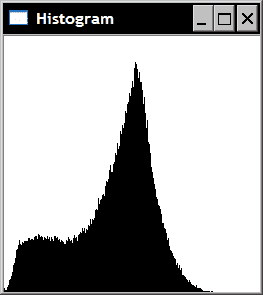cv::Mat thresholded;
cv::threshold(image,thresholded,60,255,cv::THRESH_BINARY);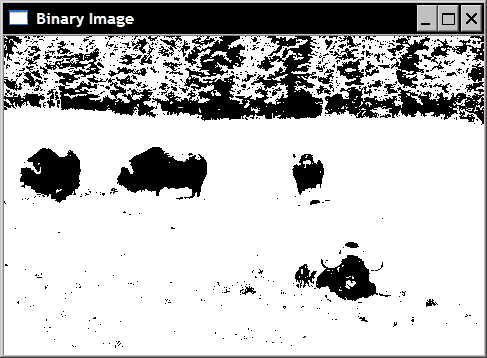## 工作原理

         float binVal = hist.at<float>(h);


getHistogramImage方法中访问 1D 直方图的每个箱子时。 注意，直方图中的值存储为float

## 更多

class ColorHistogram {

private:

int histSize;
float hranges;
const float* ranges;
int channels;

public:

ColorHistogram() {

// Prepare arguments for a color histogram
histSize= histSize= histSize= 256;
hranges= 0.0;    // BRG range
hranges= 255.0;
ranges= hranges; // all channels have the same range
ranges= hranges;
ranges= hranges;
channels= 0;      // the three channels
channels= 1;
channels= 2;
}


   cv::MatND getHistogram(const cv::Mat &image) {

cv::MatND hist;

// Compute histogram
cv::calcHist(&image,
1,            // histogram of 1 image only
channels,   // the channel used
cv::Mat(),   // no mask is used
hist,         // the resulting histogram
3,            // it is a 3D histogram
histSize,   // number of bins
ranges      // pixel value range
);

return hist;
}


   cv::SparseMat getSparseHistogram(const cv::Mat &image) {

cv::SparseMat hist(3,histSize,CV_32F);

// Compute histogram
cv::calcHist(&image,
1,            // histogram of 1 image only
channels,   // the channel used
cv::Mat(),   // no mask is used
hist,         // the resulting histogram
3,            // it is a 3D histogram
histSize,   // number of bins
ranges      // pixel value range
);

return hist;
}


# 应用查询表修改图像外观

## 操作步骤

         newIntensity= lookup[oldIntensity];


OpenCV 中的函数cv::LUT将查找表应用于图像，以生成新图像。 我们可以将此函数添加到我们的Histogram1D类中：

   cv::Mat applyLookUp(const cv::Mat& image, // input image
const cv::Mat& lookup) { // 1x256 uchar matrix

// the output image
cv::Mat result;

// apply lookup table
cv::LUT(image,lookup,result);

return result;
}


## 工作原理

   // Create an image inversion table
int dim(256);
cv::Mat lut(1,  // 1 dimension
&dim,       // 256 entries
CV_8U);     // uchar

for (int i=0; i<256; i++) {

lut.at<uchar>(i)= 255-i;
}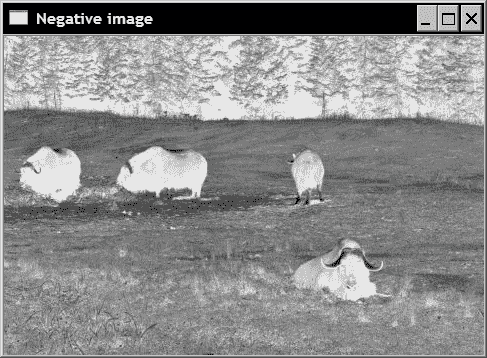## 更多

255.0*(i-imin)/(imax-imin)+0.5);


   cv::Mat stretch(const cv::Mat &image, int minValue=0) {

// Compute histogram first
cv::MatND hist= getHistogram(image);

// find left extremity of the histogram
int imin= 0;
for( ; imin < histSize; imin++ ) {
std::cout<<hist.at<float>(imin)<<std::endl;
if (hist.at<float>(imin) > minValue)
break;
}

// find right extremity of the histogram
int imax= histSize-1;
for( ; imax >= 0; imax-- ) {

if (hist.at<float>(imax) > minValue)
break;
}

// Create lookup table
int dim(256);
cv::Mat lookup(1,  // 1 dimension
&dim,       // 256 entries
CV_8U);     // uchar

// Build lookup table
for (int i=0; i<256; i++) {

// stretch between imin and imax
if (i < imin) lookup.at<uchar>(i)= 0;
else if (i > imax) lookup.at<uchar>(i)= 255;
// linear mapping
else lookup.at<uchar>(i)= static_cast<uchar>(
255.0*(i-imin)/(imax-imin)+0.5);
}

// Apply lookup table
cv::Mat result;
result= applyLookUp(image,lookup);

return result;
}


// ignore starting and ending bins with less than 100 pixels
cv::Mat streteched= h.stretch(image,100);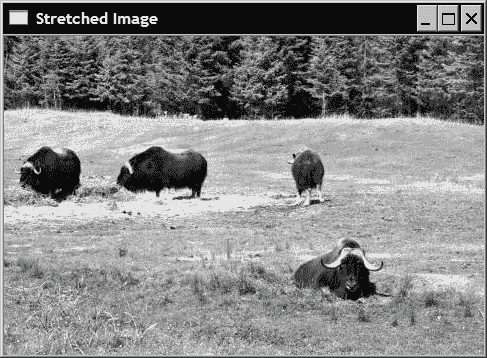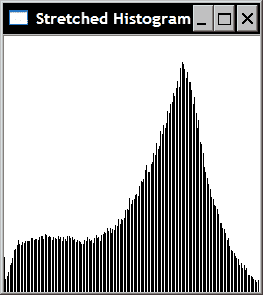## 另见

“均衡图像直方图”秘籍为您提供了另一种改善图像对比度的方法。

# 均衡图像直方图

## 操作步骤

OpenCV 提供了易于使用的函数，可以执行直方图均衡化。 可以这样调用：

   cv::Mat equalize(const cv::Mat &image) {

cv::Mat result;
cv::equalizeHist(image,result);

return result;
}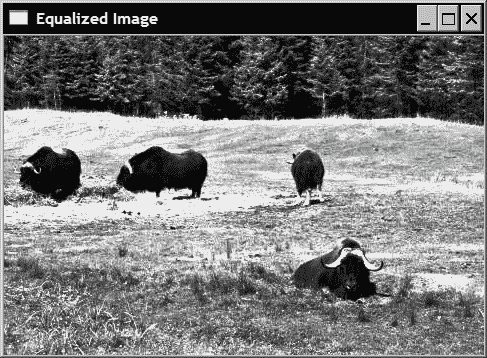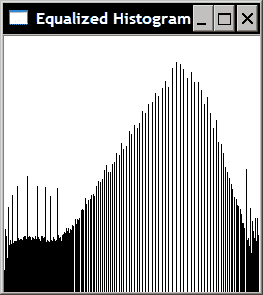## 工作原理

lookup.at<uchar>(i)= static_cast<uchar>(255.0*p[i]);


# 反投影直方图来检测特定图像内容

## 操作步骤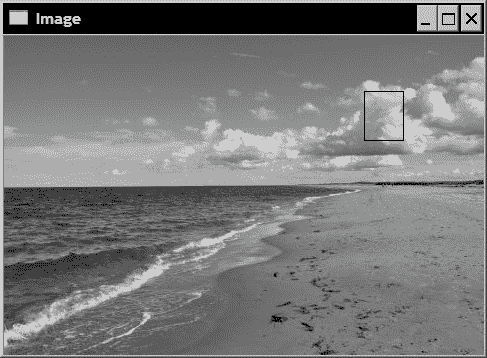cv::Mat imageROI;
imageROI= image(cv::Rect(360,55,40,50)); // Cloud region


   Histogram1D h;
cv::MatND hist= h.getHistogram(imageROI);


   cv::normalize(histogram,histogram,1.0);


cv::calcBackProject(&image,
1,            // one image
channels,     // the channels used
histogram,    // the histogram we are backprojecting
result,       // the resulting back projection image
ranges,       // the range of values, for each dimension
255.0         // a scaling factor
);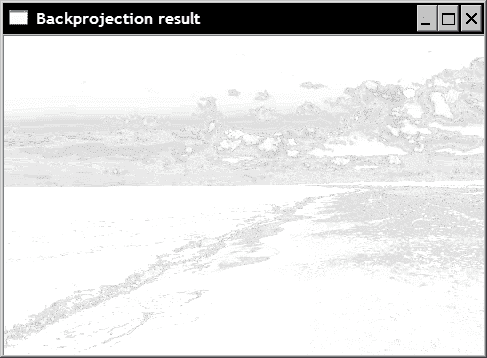cv::threshold(result, result, 255*threshold,
255, cv::THRESH_BINARY);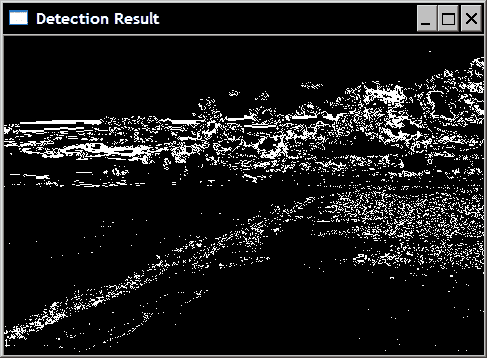## 更多

class ContentFinder {

private:

float hranges;
const float* ranges;
int channels;

float threshold;
cv::MatND histogram;

public:

ContentFinder() : threshold(-1.0f) {

ranges= hranges; // all channels have same range
ranges= hranges;
ranges= hranges;
}


   // Sets the threshold on histogram values [0,1]
void setThreshold(float t) {

threshold= t;
}

// Gets the threshold
float getThreshold() {

return threshold;
}


   // Sets the reference histogram
void setHistogram(const cv::MatND& h) {

histogram= h;
cv::normalize(histogram,histogram,1.0);
}


   cv::Mat find(const cv::Mat& image,
float minValue, float maxValue,
int *channels, int dim) {

cv::Mat result;

hranges= minValue;
hranges= maxValue;

for (int i=0; i<dim; i++)
this->channels[i]= channels[i];

cv::calcBackProject(&image, 1, // input image
channels,       // list of channels used
histogram,    // the histogram we are using
result,       // the resulting backprojection
ranges,       // the range of values
255.0         // the scaling factor
);
}

// Threshold back projection to obtain a binary image
if (threshold>0.0)
cv::threshold(result, result,
255*threshold, 255, cv::THRESH_BINARY);

return result;
}


   ColorHistogram hc;
// reduce colors
color= hc.colorReduce(color,32);
// blue sky area
cv::Mat imageROI= color(cv::Rect(0,0,165,75));


   cv::MatND hist= hc.getHistogram(imageROI);

ContentFinder finder;
finder.setHistogram(hist);
finder.setThreshold(0.05f);

// Get back-projection of color histogram
Cv::Mat result= finder.find(color);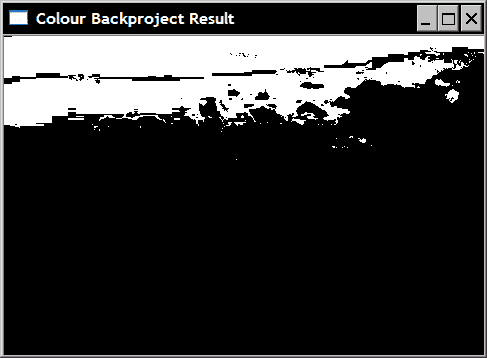# 使用均值移动算法查找对象

## 操作步骤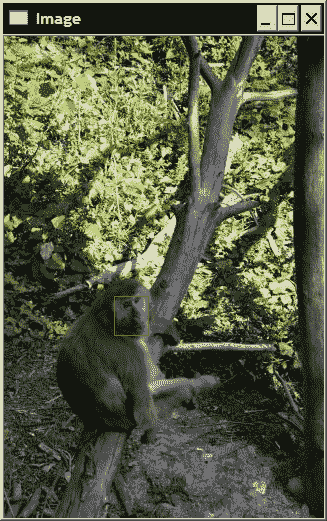// Read reference image
// Baboon's face ROI
cv::Mat imageROI= image(cv::Rect(110,260,35,40));
// Get the Hue histogram
int minSat=65;
ColorHistogram hc;
cv::MatND colorhist=
hc.getHueHistogram(imageROI,minSat);


   // Computes the 1D Hue histogram with a mask.
// BGR source image is converted to HSV
// Pixels with low saturation are ignored
cv::MatND getHueHistogram(const cv::Mat &image,
int minSaturation=0) {

cv::MatND hist;

// Convert to HSV color space
cv::Mat hsv;
cv::cvtColor(image, hsv, CV_BGR2HSV);

// Mask to be used (or not)

if (minSaturation>0) {

// Spliting the 3 channels into 3 images
std::vector<cv::Mat> v;
cv::split(hsv,v);

// Mask out the low saturated pixels
cv::THRESH_BINARY);
}

// Prepare arguments for a 1D hue histogram
hranges= 0.0;
hranges= 180.0;
channels= 0; // the hue channel

// Compute histogram
cv::calcHist(&hsv,
1,          // histogram of 1 image only
channels,   // the channel used
hist,       // the resulting histogram
1,          // it is a 1D histogram
histSize,   // number of bins
ranges      // pixel value range
);

return hist;
}


   ContentFinder finder;
finder.setHistogram(colorhist);


   image= cv::imread("../baboon3.jpg");

// Display image
cv::namedWindow("Image 2");
cv::imshow("Image 2",image);

// Convert to HSV space
cv::cvtColor(image, hsv, CV_BGR2HSV);

// Split the image
cv::split(hsv,v);

// Identify pixels with low saturation
cv::threshold(v,v,minSat,255,cv::THRESH_BINARY);


   // Get back-projection of hue histogram
result= finder.find(hsv,0.0f,180.0f,ch,1);
// Eliminate low stauration pixels
cv::bitwise_and(result,v,result);


   cv::Rect rect(110,260,35,40);
cv::rectangle(image, rect, cv::Scalar(0,0,255));

cv::TermCriteria criteria(cv::TermCriteria::MAX_ITER,
10,0.01);
cv::meanShift(result,rect,criteria);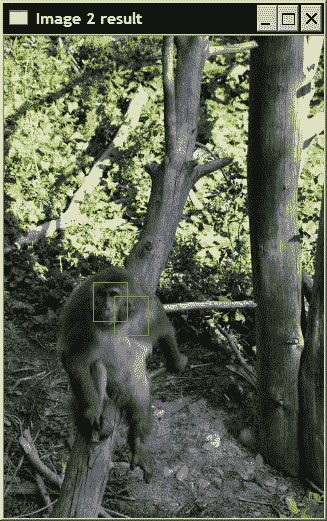## 另见

OpenCV 还提供了 CamShift 算法的实现，该算法是均值偏移的改进版本，其中窗口的大小和方向可以更改。

# 使用直方图比较检索相似的图像

## 操作步骤

class ImageComparator {

private:

cv::Mat reference;
cv::Mat input;
cv::MatND refH;
cv::MatND inputH;

ColorHistogram hist;
int div;

public:

ImageComparator() : div(32) {

}


   // Color reduction factor
// The comparison will be made on images with
// color space reduced by this factor in each dimension
void setColorReduction( int factor) {

div= factor;
}

int getColorReduction() {

return div;
}


   void setReferenceImage(const cv::Mat& image) {

reference= hist.colorReduce(image,div);
refH= hist.getHistogram(reference);
}


   double compare(const cv::Mat& image) {

input= hist.colorReduce(image,div);
inputH= hist.getHistogram(input);

return cv::compareHist(
refH,inputH,CV_COMP_INTERSECT);
}
};


   ImageComparator c;
c.setReferenceImage(image);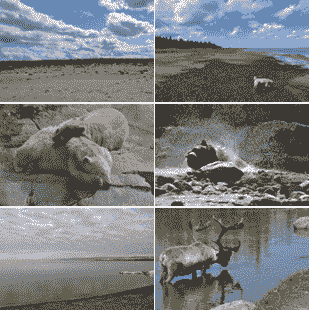## 工作原理

cv::compareHist的调用非常简单。 您只需输入两个直方图，函数就会返回测得的距离。 使用标志指定要使用的特定测量方法。 在ImageComparator类中，使用相交方法（带有标志CV_COMP_INTERSECT）。 此方法仅针对每个箱子比较每个直方图中的两个值，并保持最小值。 那么，相似性度量只是这些最小值的总和。 因此，具有没有共同颜色的直方图的两个图像的相交值将为 0，而两个相同直方图的值将等于像素总数。

## 另见

OpenCV 文档描述了不同直方图比较度量中使用的确切公式。